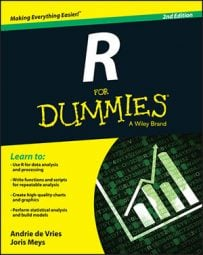##### R For DummiesYou probably are itching to get started on some real R code. Here, you get to do exactly that. Get ready to get your hands dirty and dive into the programming world!

## Saying hello to the world

Programming books typically start with a very simple program. Often, this first program creates the message Hello world!. In R, hello world program consists of one line of code.

Start a new R session, type the following in your console, and press Enter:

`> print("Hello world!")`

R responds immediately with this output:

` "Hello world!"`

You can collapse input and output into a single block of code, like this:

```> print("Hello world!")
 "Hello world!"```

## Doing simple math

Type the following in your console to calculate the sum of five numbers:

```> 1 + 2 + 3 + 4 + 5
 15```

The answer is 15, which you can easily verify for yourself. You may think that there’s an easier way to calculate this value, though — and you’d be right.

## Using vectors

A vector is the simplest type of data structure in R. The R manual defines a vector as “a single entity consisting of a collection of things”. A collection of numbers, for example, is a numeric vector — the first five integer numbers form a numeric vector of length 5.

To construct a vector, type into the console:

```> c(1, 2, 3, 4, 5)
 1 2 3 4 5```

In constructing your vector, you have successfully used a function in R. In programming language, a function is a piece of code that takes some inputs and does something specific with them. In constructing a vector, you tell the c() function to construct a vector with the first five integers. The entries inside the parentheses are referred to as arguments.

You also can construct a vector by using operators. An operator is a symbol you stick between two values to make a calculation. The symbols +, -, *, and / are all operators, and they have the same meaning they do in mathematics. Thus, 1+2 in R returns the value 3, just as you’d expect.

One very handy operator is called sequence, and it looks like a colon (:). Type the following in your console:

```> 1:5
 1 2 3 4 5```

That’s more like it. With three keystrokes, you’ve generated a vector with the values 1 through 5. To calculate the sum of this vector, type into your console:

```> sum(1:5)
 15```

While quite basic, this example shows you that using vectors allows you to do complex operations with a small amount of code.

## Storing and calculating values

Using R as a calculator is very interesting but perhaps not all that useful. A much more useful capability is storing values and then doing calculations on these stored values. Try this:

```> x <- 1:5
> x
 1 2 3 4 5```

In these two lines of code, you first assign the sequence 1:5 to an object called x. Then you ask R to print the value of x by typing x in the console and pressing Enter.

In R, the assignment operator is <-, which you type in the console by using two keystrokes: the less-than symbol (<) followed by a hyphen (-). The combination of these two symbols represents assignment. It’s good practice to always surround the <- with spaces. This makes your code much easier to read and understand.

In addition to retrieving the value of a variable, you can do calculations on that value. Create a second variable called y, and assign it the value 10. Then add the values of x and y, as follows:

```> y <- 10
> x + y
 11 12 13 14 15```

The values of the two variables themselves don’t change unless you assign a new value to either of them. You can check this by typing the following:

```> x
 1 2 3 4 5
> y
 10```

Now create a new variable z, assign it the value of x + y, and print its value:

```> z <- x + y
> z
 11 12 13 14 15```

Variables also can take on text values. You can assign the value Hello to a variable called h, for example, by presenting the text to R inside quotation marks, like this:

```> h <- "Hello"
> h
 "Hello"```

You must enter text or character values to R inside quotation marks — either single or double. R accepts both. So both h <- Hello and h <- Hello are examples of valid R syntax.

You can use the c() function to combine numeric values into vectors. This technique also works for text:

```> hw <- c("Hello", "world!")
> hw
 "Hello" "world!"```

You use the paste() function to concatenate multiple text elements. By default, paste() puts a space between the different elements, like this:

```> paste("Hello", "world!")
 "Hello world!"```

## Talking back to the user

You can write R scripts that have some interaction with a user. To ask the user questions, you can use the readline() function. In the following code snippet, you read a value from the keyboard and assign it to the variable yourname:

```> h <- "Hello"
> paste(h, yourname)
 "Hello Andrie"```

This code seems to be a bit cumbersome, however. Clearly, it would be much better to send these three lines of code simultaneously to R and get them evaluated in one go.